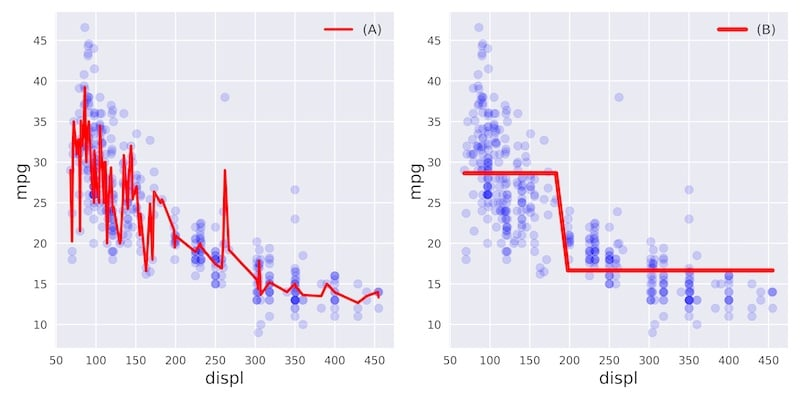# Overfitting and underfitting

In this exercise, you'll visually diagnose whether a model is overfitting or underfitting the training set.

For this purpose, we have trained two different models $$A$$ and $$B$$ on the auto dataset to predict the mpg consumption of a car using only the car's displacement (displ) as a feature.

The following figure shows you scatterplots of mpg versus displ along with lines corresponding to the training set predictions of models $$A$$ and $$B$$ in red.Which of the following statements is true?